Advertisement Remove all ads

# NCERT solutions for Class 9 Mathematics Textbook chapter 9 - Areas of Parallelograms and Triangles [Latest edition]

#### Chapters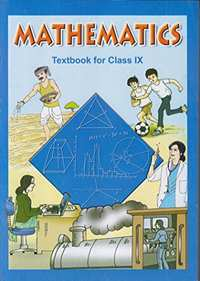Advertisement Remove all ads
Advertisement Remove all ads
Advertisement Remove all ads

## Chapter 9: Areas of Parallelograms and Triangles

Exercise 9.1Exercise 9.2Exercise 9.3Exercise 9.4
Advertisement Remove all ads
Exercise 9.1[Page 155]

### NCERT solutions for Class 9 Mathematics Textbook Chapter 9 Areas of Parallelograms and TrianglesExercise 9.1[Page 155]

Exercise 9.1 | Q 1 | Page 155

Which of the following figures lie on the same base and between the same parallels. In such a case, write the common base and the two parallels.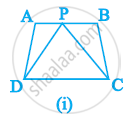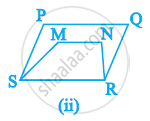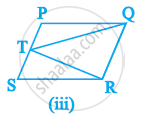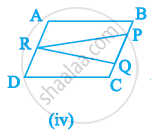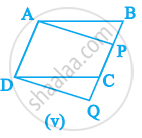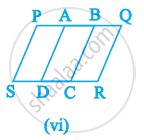Exercise 9.2[Page 159]

### NCERT solutions for Class 9 Mathematics Textbook Chapter 9 Areas of Parallelograms and TrianglesExercise 9.2[Page 159]

Exercise 9.2 | Q 1 | Page 159

In the given figure, ABCD is parallelogram, AE ⊥ DC and CF ⊥ AD. If AB = 16 cm, AE = 8 cm and CF = 10 cm, find AD.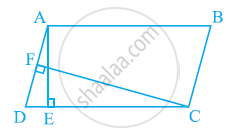Exercise 9.2 | Q 2 | Page 159

If E, F, G and H are respectively the mid-points of the sides of a parallelogram ABCD show that ar (EFGH) = 1/2ar (ABCD)

Exercise 9.2 | Q 3 | Page 159

P and Q are any two points lying on the sides DC and AD respectively of a parallelogram ABCD. Show that ar (APB) = ar (BQC).

Exercise 9.2 | Q 4 | Page 159

In the given figure, P is a point in the interior of a parallelogram ABCD. Show that

(i) ar (APB) + ar (PCD) = 1/2ar (ABCD)

(ii) ar (APD) + ar (PBC) = ar (APB) + ar (PCD)

[Hint: Through. P, draw a line parallel to AB]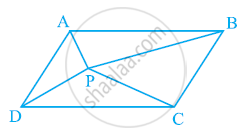Exercise 9.2 | Q 5 | Page 159

In the given figure, PQRS and ABRS are parallelograms and X is any point on side BR. Show that

(i) ar (PQRS) = ar (ABRS)

(ii) ar (AXS) = 1/2ar (PQRS)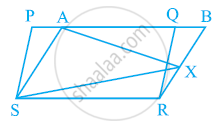Exercise 9.2 | Q 6 | Page 159

A farmer was having a field in the form of a parallelogram PQRS. She took any point A on RS and joined it to points P and Q. In how many parts the field is divided? What are the shapes of these parts? The farmer wants to sow wheat and pulses in equal portions of the field separately. How should she do it?

Exercise 9.3[Pages 162 - 164]

### NCERT solutions for Class 9 Mathematics Textbook Chapter 9 Areas of Parallelograms and TrianglesExercise 9.3[Pages 162 - 164]

Exercise 9.3 | Q 1 | Page 162

In the given figure, E is any point on median AD of a ΔABC. Show that ar (ABE) = ar (ACE)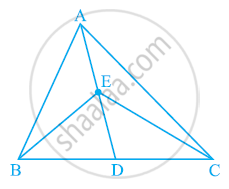Exercise 9.3 | Q 2 | Page 162

In a triangle ABC, E is the mid-point of median AD. Show that ar (BED) = 1/4ar (ABC).

Exercise 9.3 | Q 3 | Page 162

Show that the diagonals of a parallelogram divide it into four triangles of equal area.

Exercise 9.3 | Q 4 | Page 162

In the given figure, ABC and ABD are two triangles on the same base AB. If line-segment CD is bisected by AB at O, show that ar (ABC) = ar (ABD).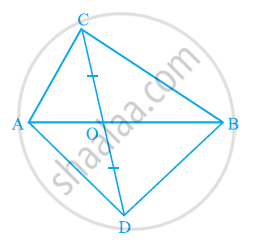Exercise 9.3 | Q 5 | Page 163

D, E and F are respectively the mid-points of the sides BC, CA and AB of a ΔABC. Show that

(i) BDEF is a parallelogram.

(ii) ar (DEF) = 1/4ar (ABC)

(iii) ar (BDEF) = 1/2ar (ABC)

Exercise 9.3 | Q 6 | Page 163

In the given figure, diagonals AC and BD of quadrilateral ABCD intersect at O such that OB = OD. If AB = CD, then show that:

(i) ar (DOC) = ar (AOB)

(ii) ar (DCB) = ar (ACB)

(iii) DA || CB or ABCD is a parallelogram.

[Hint: From D and B, draw perpendiculars to AC.]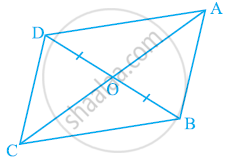Exercise 9.3 | Q 7 | Page 163

D and E are points on sides AB and AC respectively of ΔABC such that

ar (DBC) = ar (EBC). Prove that DE || BC.

Exercise 9.3 | Q 8 | Page 163

XY is a line parallel to side BC of a triangle ABC. If BE || AC and CF || AB meet XY at E and F respectively, show that

ar (ABE) = ar (ACF)

Exercise 9.3 | Q 9 | Page 163

The side AB of a parallelogram ABCD is produced to any point P. A line through A and parallel to CP meets CB produced at Q and then parallelogram PBQR is completed (see the following figure). Show that

ar (ABCD) = ar (PBQR).

[Hint: Join AC and PQ. Now compare area (ACQ) and area (APQ)]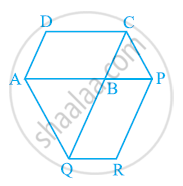Exercise 9.3 | Q 10 | Page 163

Diagonals AC and BD of a trapezium ABCD with AB || DC intersect each other at O. Prove that ar (AOD) = ar (BOC).

Exercise 9.3 | Q 11 | Page 163

In the given figure, ABCDE is a pentagon. A line through B parallel to AC meets DC produced at F. Show that

(i) ar (ACB) = ar (ACF)

(ii) ar (AEDF) = ar (ABCDE)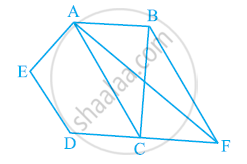Exercise 9.3 | Q 12 | Page 164

A villager Itwaari has a plot of land of the shape of a quadrilateral. The Gram Panchayat of the village decided to take over some portion of his plot from one of the corners to construct a Health Centre. Itwaari agrees to the above proposal with the condition that he should be given equal amount of land in lieu of his land adjoining his plot so as to form a triangular plot. Explain how this proposal will be implemented.

Exercise 9.3 | Q 13 | Page 164

ABCD is a trapezium with AB || DC. A line parallel to AC intersects AB at X and BC at Y. Prove that ar (ADX) = ar (ACY).

[Hint: Join CX.]

Exercise 9.3 | Q 14 | Page 164

In the given figure, AP || BQ || CR. Prove that ar (AQC) = ar (PBR).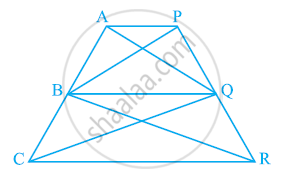Exercise 9.3 | Q 15 | Page 164

Diagonals AC and BD of a quadrilateral ABCD intersect at O in such a way that ar (AOD) = ar (BOC). Prove that ABCD is a trapezium.

Exercise 9.3 | Q 16 | Page 164

In the given figure, ar (DRC) = ar (DPC) and ar (BDP) = ar (ARC). Show that both the quadrilaterals ABCD and DCPR are trapeziums.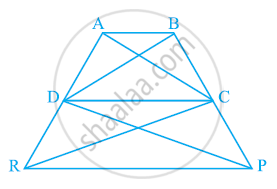Exercise 9.4[Pages 164 - 166]

### NCERT solutions for Class 9 Mathematics Textbook Chapter 9 Areas of Parallelograms and TrianglesExercise 9.4[Pages 164 - 166]

Exercise 9.4 | Q 1 | Page 164

Parallelogram ABCD and rectangle ABEF are on the same base AB and have equal areas. Show that the perimeter of the parallelogram is greater than that of the rectangle.

Exercise 9.4 | Q 2 | Page 164

In the following figure, D and E are two points on BC such that BD = DE = EC. Show that ar (ABD) = ar (ADE) = ar (AEC).

Can you answer the question that you have left in the ’Introduction’ of this chapter, whether the field of Budhia has been actually divided into three parts of equal area?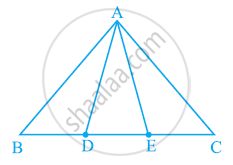[Remark: Note that by taking BD = DE = EC, the triangle ABC is divided into three triangles ABD, ADE and AEC of equal areas. In the same way, by dividing BC into n equal parts and joining the points of division so obtained to the opposite vertex of BC, you can divide ΔABC into n triangles of equal areas.]

Exercise 9.4 | Q 3 | Page 165

In the following figure, ABCD, DCFE and ABFE are parallelograms. Show that ar (ADE) = ar (BCF).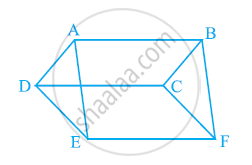Exercise 9.4 | Q 4 | Page 165

In the following figure, ABCD is parallelogram and BC is produced to a point Q such that AD = CQ. If AQ intersect DC at P, show that

ar (BPC) = ar (DPQ).

[Hint: Join AC.]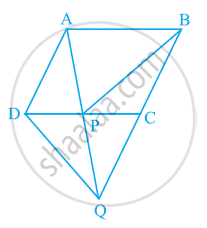Exercise 9.4 | Q 5 | Page 165

In the following figure, ABC and BDE are two equilateral triangles such that D is the mid-point of BC. If AE intersects BC at F, show that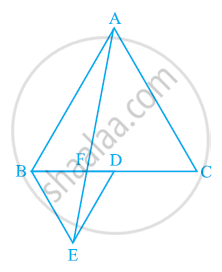(i) ar (BDE) = 1/4 ar (ABC)

(ii) ar (BDE) = 1/2 ar (BAE)

(iii) ar (ABC) = 2 ar (BEC)

(iv) ar (BFE) = ar (AFD)

(v) ar (BFE) = 2 ar (FED)

(vi) ar (FED) = 1/8 ar (AFC)

[Hint : Join EC and AD. Show that BE || AC and DE || AB, etc.]

Exercise 9.4 | Q 6 | Page 166

Diagonals AC and BD of a quadrilateral ABCD intersect each other at P. Show that ar (APB) × ar (CPD) = ar (APD) × ar (BPC).

[Hint : From A and C, draw perpendiculars to BD.]

Exercise 9.4 | Q 7 | Page 166

P and Q are respectively the mid-points of sides AB and BC of a triangle ABC and R is the mid-point of AP, show that

(i) ar(PRQ) = 1/2 ar(ARC)

(ii) ar(RQC) = 3/8 ar(ABC)

(iii) ar(PBQ) = ar(ARC)

Exercise 9.4 | Q 8 | Page 166

In the following figure, ABC is a right triangle right angled at A. BCED, ACFG and ABMN are squares on the sides BC, CA and AB respectively. Line segment AX ⊥ DE meets BC at Y. Show that:-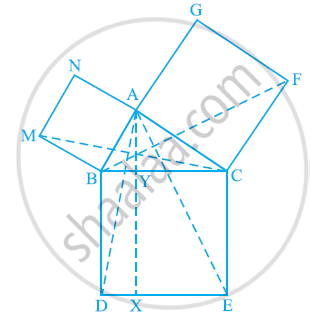(i) ΔMBC ≅ ΔABD

(ii) ar (BYXD) = 2 ar(MBC)

(iii) ar (BYXD) = ar(ABMN)

(iv) ΔFCB ≅ ΔACE

(v) ar(CYXE) = 2 ar(FCB)

(vi) ar (CYXE) = ar(ACFG)

(vii) ar (BCED) = ar(ABMN) + ar(ACFG)

Note : Result (vii) is the famous Theorem of Pythagoras. You shall learn a simpler proof of this theorem in Class X.

## Chapter 9: Areas of Parallelograms and Triangles

Exercise 9.1Exercise 9.2Exercise 9.3Exercise 9.4## NCERT solutions for Class 9 Mathematics Textbook chapter 9 - Areas of Parallelograms and Triangles

NCERT solutions for Class 9 Mathematics Textbook chapter 9 (Areas of Parallelograms and Triangles) include all questions with solution and detail explanation. This will clear students doubts about any question and improve application skills while preparing for board exams. The detailed, step-by-step solutions will help you understand the concepts better and clear your confusions, if any. Shaalaa.com has the CBSE Class 9 Mathematics Textbook solutions in a manner that help students grasp basic concepts better and faster.

Further, we at Shaalaa.com provide such solutions so that students can prepare for written exams. NCERT textbook solutions can be a core help for self-study and acts as a perfect self-help guidance for students.

Concepts covered in Class 9 Mathematics Textbook chapter 9 Areas of Parallelograms and Triangles are Corollary: Triangles on the same base and between the same parallels are equal in area., Corollary: A rectangle and a parallelogram on the same base and between the same parallels are equal in area., Theorem: Parallelograms on the Same Base and Between the Same Parallels., Concept of Area.

Using NCERT Class 9 solutions Areas of Parallelograms and Triangles exercise by students are an easy way to prepare for the exams, as they involve solutions arranged chapter-wise also page wise. The questions involved in NCERT Solutions are important questions that can be asked in the final exam. Maximum students of CBSE Class 9 prefer NCERT Textbook Solutions to score more in exam.

Get the free view of chapter 9 Areas of Parallelograms and Triangles Class 9 extra questions for Class 9 Mathematics Textbook and can use Shaalaa.com to keep it handy for your exam preparation

Advertisement Remove all ads
Share
Notifications

View all notifications

Forgot password?